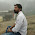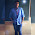# Hackerrank Solution For Day 1: Data Types

Day 1: Data Types Problem Statement- Write a hackerrank solution for day 1: data types or hacker rank solution program in c++ for " day 1: data types " or hackerrank solution for 30 days of code challenges.

Logic:- So here is a logic of problem Hackerrank Solution For Day Day 1: Data Types. Here in this problem some data type is already defined they are following, int, double, string and we have to initialize another data type which is same int, double, and string for the first data type int we have to perform addition with user input data. and for double also we have to repeat the same process. and for string first we have to print the already initialize string that is "HackerRank " and after the "HackerRank " user string will be shown. for this Day 1: Data Types solution I am going to explain program step by step.

### Data Types Solution Explanation

as we know that int 1 = 4; are already define so we have to take another integer j, after that we will take user input and store the value in j then we will add i + j value like below.

int i = 4;
int j = 12;
cout << i + j <<endl;

So the i + j value will be added and print the sum of both numbers.

Now repeat the same process for double data type so add both double value fixed value and user input value and print the output but remember as you know that double-precision value is more than 1 so this is a problem for avoiding this type of problem we can fix the double value by using set precision(value) means how many digits you want after pointer and fraction number. Here you can see that we fixed value to one that's we use set precision(1).

double d = 4.0;
double e = 4.0;
cout<<fixed<<setprecision(1)<<d<<endl;

Now next step is for the string is very simple we can print the first string and then user input sting but we have one problem we can not accept space user input string so for that we use getline(cin,t). if you don't know about getline() function see the example. Hackerrank Solution For Day 0: Hello, World.

string s = " HackerRank ";
string t ; // user input.
cout<<s<<t;

I hope now you can understand a full problem if you have any query send an email. You can directly submit your solution copy the coloured area code and paste into Hacker Rank Editor.

Updated Solutions: Day 1 Data Types Solution in C, C++, and Java Programming Language

## Hackerrank Day 1: Data Types Solution

#include <iostream>
#include <iomanip>
#include <limits>

using namespace std;

int main()
{
int i = 4;
double d = 4.0;
string s = "HackerRank ";

int j;
double e;
string t;

cin>>j;
cin>>e;

cin.ignore();
getline(cin,t);

cout<<i+j<<endl;

d=d+e;

cout<<fixed<<setprecision(1)<<d<<endl;

cout<<s<<t;

return 0;
}

### Similar on 30 Days of Code

1. Day 2: Operators Solution

2. Day 3: Intro to Conditional Statements Solution

3. Class vs. Instance Solution

4. Hackerrank Day 5: Loops Solution

5. Let's Review Day 6 Solution

1.pls give code in c

2.pls give code in c

1.Please send your mail ID i will send you code in C language

3.for what cin.ignore(); function is used

1.When you use getline() instead of cin, and take a input in next line at that time for clear buffer or avoiding a stream input error. The particular function skips the next input character or to skip the characters until a newline character is read.

4.plz send me code in c
this is my mail id dholendar1286@gmail.com

1.Hello, venkatasai dholendar

I already sent your solution . Check your Email, Thanks for Visiting Keep Share and Keep Visit.

2.Here is a Solution In C Language. May be Header files can be problem in comment section. So please add all necessary header files.

Solution:-

#include
#include
#include
#include

int main()
{
int i = 4;
double d = 4.0;
char s[] = "HackerRank ";

int j;
double e;
char t;

scanf("%d",&j);
scanf("%lf",&e);

scanf(" %[^\n]s",t);
printf("%d\n",(i+j));

printf("%.1lf\n",(e+d));

printf("%s",s);
printf("%s",t);

return 0;
}

3.Why did you use .1lf

4.Please send me the solutions in c

5.cin.ignore();
what is its use

6.Please send me the solutions in c